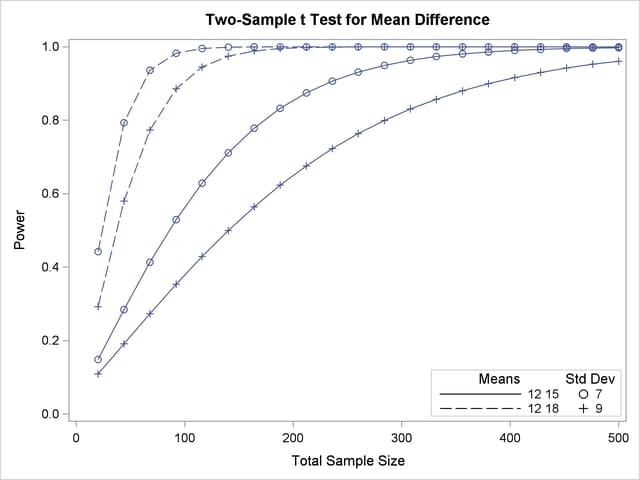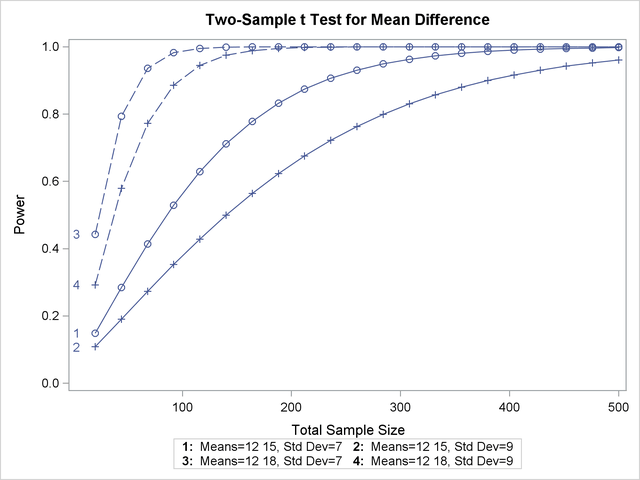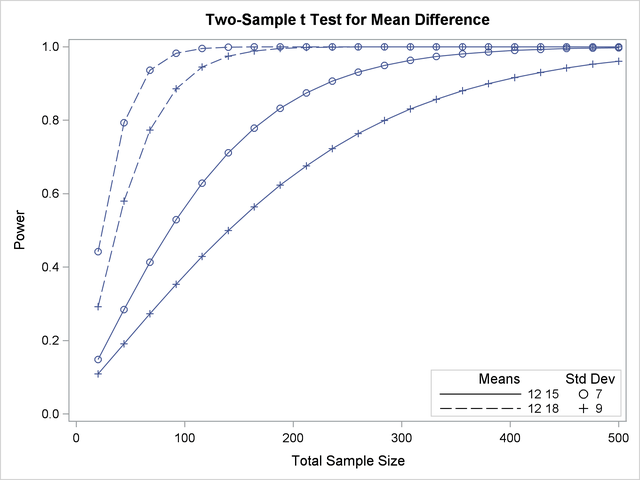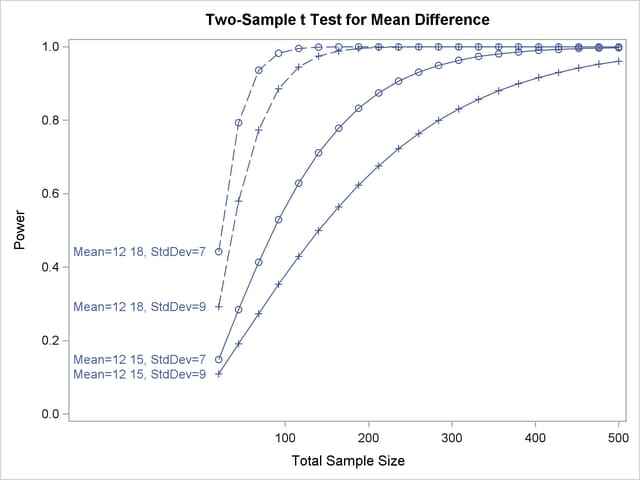### Choosing Key (Legend) Styles

The default style for the key (or "legend") is one that displays the association between levels of features and levels of analysis parameters, located below the X axis. For example, Output 70.8.5 demonstrates this style of key.

You can reproduce Output 70.8.5 with the same key but a different location, inside the plotting region, by using the POS=INSET option within the KEY=BYFEATURE option in the PLOT statement. The following statements product the plot in Output 70.8.17:

```proc power plotonly;
twosamplemeans test=diff
groupmeans   = 12 | 15 18
stddev       = 7 9
power        = .
ntotal       = 200;
plot x=n min=20 max=500
key = byfeature(pos=inset);
run;
```

Output 70.8.17 Plot with a By-Feature Key inside the Plotting RegionAlternatively, you can specify a key that identifies each individual curve separately by number by using the KEY=BYCURVE option in the PLOT statement:

```   plot x=n min=20 max=500
key = bycurve;
```

The resulting plot is shown in Output 70.8.18.

Output 70.8.18 Plot with a Numbered By-Curve KeyUse the NUMBERS=OFF option within the KEY=BYCURVE option to specify a nonnumbered key that identifies curves with samples of line styles, symbols, and colors:

```   plot x=n min=20 max=500
key = bycurve(numbers=off pos=inset);
```

The POS=INSET suboption places the key within the plotting region. The resulting plot is shown in Output 70.8.19.

Output 70.8.19 Plot with a Nonnumbered By-Curve KeyFinally, you can attach labels directly to curves with the KEY=ONCURVES option. The following PLOT statement produces Output 70.8.20:

```   plot x=n min=20 max=500
key = oncurves;
```

Output 70.8.20 Plot with Directly Labeled Curves